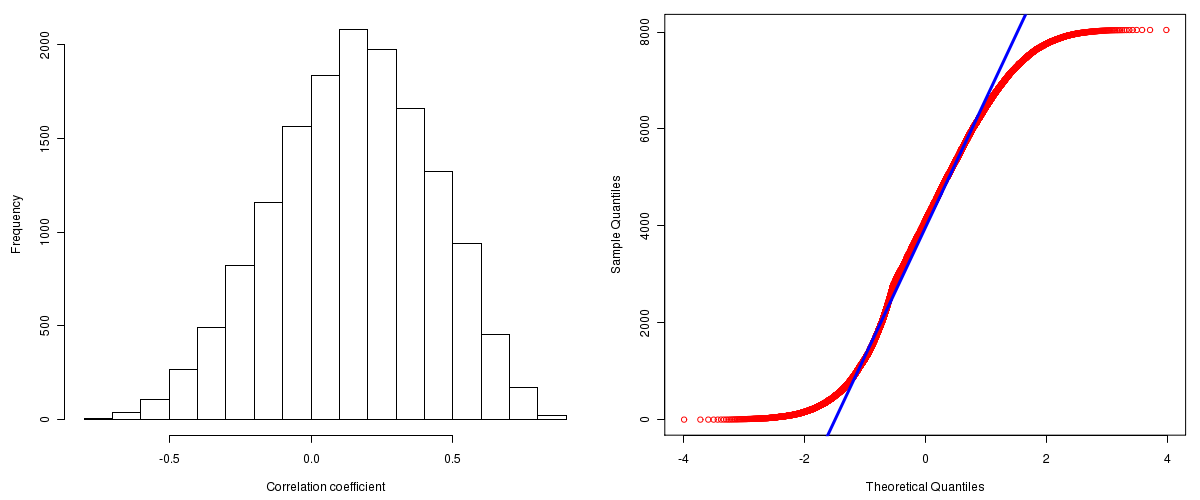Kidney Renal Clear Cell Carcinoma: Correlations between copy number and mRNA expression
Maintained by John Zhang (Dana-Farber Cancer Institute)
Overview
Introduction

A TCGA sample is profiled to detect the copy number variations and expressions of genes. This pipeline attempts to correlate copy number and expression data of genes across samples to determine if the copy number variations also result in differential expressions. This report contains the calculated correlation coefficients based on measurements of genomic copy number (log2) values and intensity of the expressions of the corresponding feature across patients. High positive/low negative correlation coefficients indicate that genomic alterations result in differences in the expressions of mRNA the genomic regions transcribe.

Summary

The correlation coefficients in 10, 20, 30, 40, 50, 60, 70, 80, 90 percentiles are -0.2254, -0.09288, 0.00138, 0.083, 0.1566, 0.2295, 0.30552, 0.3963, 0.50862, respectively.

Results
Correlation results

Number of genes and samples used for the calculation are shown in Table 1. Figure 1 shows the distribution of calculated correlation coefficients and quantile-quantile plot of the calculated correlation coefficients against a normal distribution. Table 2 shows the top 20 features ordered by the value of correlation coefficients.

Table 1.  Counts of mRNA and number of samples in copy number and expression data sets and common to both

Category Copy number Expression Common
Sample 489 72 28
Genes 29390 17815 15551

Figure 1.  Summary figures. Left: histogram showing the distribution of the calculated correlations across samples for all Genes. Right: QQ plot of the calculated correlations across samples. The QQ plot is used to plot the quantiles of the calculated correlation coefficients against that derived from a normal distribution. Points deviating from the blue line indicate deviation from normality.Table 2.  Get Full Table Top 20 features (defined by the feature column) ranked by correlation coefficients

feature r p-value q-value chrom start end geneid
ZNF193 0.8617 3.87569132342946e-09 1.76755455982858e-05 6 28301049 28309239 7746
SFRS11 0.8582 5.28199350924297e-09 1.76755455982858e-05 1 70443953 70490272 9295
ABCF2 0.8539 7.59334728428485e-09 1.76755455982858e-05 7 150535856 150555250 10061
CDC5L 0.8525 8.49254178092451e-09 1.76755455982858e-05 6 44463280 44522758 988
ACVR1 0.8498 1.06166941904462e-08 1.76772388862699e-05 2 158301207 158403036 90
FASTK 0.8464 1.38220925816057e-08 1.91786341468591e-05 7 150404641 150408884 10922
IFI44L 0.8408 2.13081501332368e-08 2.47229880050741e-05 1 78858741 78880657 10964
FBXO42 0.8387 2.49670097929311e-08 2.47229880050741e-05 1 16449146 16551535 54455
PRPF38A 0.8378 2.6726884705397e-08 2.47229880050741e-05 1 52642824 52656438 84950
IFI44 0.8335 3.65083694386925e-08 3.03939793470136e-05 1 78888104 78902345 10561
RBM16 0.828 5.41371290019299e-08 4.09729904798558e-05 6 155096204 155196886 22828
LARS 0.8263 6.0911303334521e-08 4.22582758422469e-05 5 145473221 145542321 51520
CASP8AP2 0.8195 9.59381056819808e-08 6.14388267997306e-05 6 90596379 90640876 9994
NOL7 0.8106 1.70457124859524e-07 0.000101363652307409 6 13723538 13729106 51406
GNL2 0.8083 1.96882159242406e-07 0.000106305324921356 1 37805004 37834083 29889
PHACTR4 0.8075 2.06749824593899e-07 0.000106305324921356 1 28637269 28699407 65979
KPNA6 0.8067 2.17074501929204e-07 0.000106305324921356 1 32346244 32411380 23633
LOC440087 0.8038 2.57819914528667e-07 0.000119244699197478 12 14848851 14858383 440087
GTF2E2 0.8008 3.0861987010411e-07 0.00013308781079944 8 30555573 30635274 2961
MAD2L1BP 0.8002 3.19722462727157e-07 0.00013308781079944 6 43705257 43716667 9587
Methods & Data
Input

Gene level (TCGA Level III) expression data and copy number data of the corresponding loci derived by using the CNTools package of Bioconductor were used for the calculations. Pearson correlation coefficients were calculated for each pair of genes shared by the two data sets across all the samples that were common.

Correlation across sample

Pairwise correlations between the log2 copy numbers and expressions of each gene across samples were calculated using Pearson correlation.